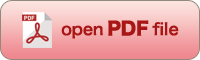[OPTICAL REVIEW Vol. 8, No. 1 (2001) 43-53]

A Simulation of Near Field Optics by Three Dimensional Volume Integral Equation of Classical Electromagnetic Theory

Kazuo TANAKA, Mengyun YAN and Masahiro TANAKA

Department of Information Science, Gifu University, Yanagido 1-1, Gifu, 501-1193 Japan

(Received June 19, 2000; Accepted November 8, 2000)A numerical simulation code for three dimensional problems of near-field optics has been developed using the volume integral equation with the moment method. The object is assumed to be continuous and macroscopic dielectric and can be treated by macroscopic Maxwell's equations. The code can treat the large-scale moment method matrix that is obtained by the discretization of the volume integral equation. The resultant matrix equation is solved by an iteration method called the generalized minimum residual method with reasonable computational cost for simple problems of near field optics. Simulation of a simplified model of a scanning near-field optical microscope has been performed and basic polarization characteristics of the system have been investigated in detail. The code is also applied to the collection-mode of a photon scanning tunneling microscope, where the incident wave is the evanescent wave, and basic relation between near-field and far field i.e., output image, is recognized.

Key words : near-field optics, three dimension, numerical simulation, volume integral equation, moment method, iteration techniques, moment method